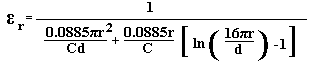<

# Relative Dielectric Constant Calculator for a Circular Capacitor

The relative dielectric constant (εr) of the dielectric in a parallel plate, circular capacitor can be calculated using the equation below.

 INPUT DATA Enter the value of the capacitor: pF Enter the radius of the circle: cm Enter the thickness of the dielectric: mm
 RESULTS Relative Dielectric Constant (εr):

This calculator uses JavaScript and will function in most modern browsers. For more information see About our calculators.

The calculator uses a derivation of Kirchhoff's equation for a circular capacitor and is intended for use with the Accurate Method for Measuring Relative Dielectric Constant of a PCB Using a Vector Network AnalyserWhere:

r is the Radius in cm

C is the Capacitance in pico Farads

d is the Dielectric thickness in cm

This calculator is provided free by Chemandy Electronics in order to promote the FLEXI-BOX